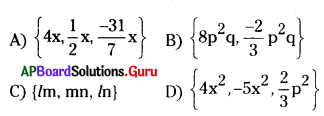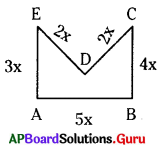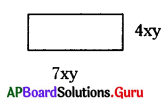Practice the AP 7th Class Maths Bits with Answers Chapter 10 Algebraic Expressions on a regular basis so that you can attempt exams with utmost confidence.

## AP State Syllabus 7th Class Maths Bits 10th Lesson Algebraic Expressions with Answers

Question 1.
The literal coefficient of-y is
A) 1
B) -1
C) y
D) -y
C) y

Question 2.
The numerical coefficient of -3z is
A) -3
B) 3
C) z
D) -z
A) -3Question 3.
No. of terms in the expression (19 × 5) ÷ 16
A) 1
B) 2
C) 3
D) 4
A) 1

Question 4.
Which of the following is a numerical expression?
A) 1 + 2 – 9
B) 9 + (6 – 4)
C) -3 – 5
D) x – $$\frac {11}{3}$$
D) x – $$\frac {11}{3}$$

Question 5.
Which of the following is a binomial?
A) 19 × 4
B) 7xyz
C) x – 4a
D) 3x + y – z
C) x – 4a

Question 6.
The degree of the monomial 9xy2z3 is
A) 7
B) 6
C) 5
D) 9
B) 6

Question 7.
The degree of the algebraic expression ax2 + bx + c is
A) 2
B) 1
C) 3
D) 4
A) 2

Question 8.
Sum of 18ab,-5ab, 12ab is
A) 25ab
B) 26ab
C) 30ab
D) 20ab
A) 25ab

Question 9.
Subtract 6x2y from 4x2y
A) -2x2y
B) 2x2y
C) 10x2y
D) -10x2y
A) -2x2yQuestion 10.
Which of the following are like terms?
A) { – 2 xy2,5y2 x}
B) {7p, – 2p, 3p}
C) {4 xyz, – 5x2 yz}
D) {lmn2, l2mn. lm2n}
B) {7p, – 2p, 3p}

Question 11.
Which of the following are not like terms?C) {lm, mn, ln}

Question 12.
Which of the following is a Numerical Expression?
A) xy – z2 + yz
B) 2 + m + n
C) 9 + 11a2 – 5b2
D) 11 ÷ (5 – 6) + 2
D) 11 ÷ (5 – 6) + 2

Question 13.
Which of the following is Algebraic Expression?
A) 96
B) 95 ÷ 11 × 3
C) 2 + m + n
D) – 30 + (16 ÷ 4)
C) 2 + m + n

Question 14.
The degree of a monomial $$\frac {-5}{2}$$ xy2 z3 is?
A) 7
B) 2
C) 3
D) 6
D) 6

Question 15.
The degree of a monomial $$\frac {-15}{19}$$ l3m4n is …………….
A) 8
B) 7
C) 9
D) 12
A) 8

Question 16.
The degree of an algebraic expression ax3 + bx2 + cx + dx11 + ex9 is ……………..
A) 11
B) 9
C) 3
D) 16
A) 11Question 17.
The degree of an algebraic expression lmn + l2m-ln2-m2n is ………………….
A) 2
B) 3
C) 6
D) 8
B) 3

Question 18.
From the given figure the perimeter is …………….. units.A) 8x
B) 10x
C) 16x
D) 12x
C) 16x

Question 19.
The perimeter of a rectangle is ……………… units.A) 11xy
B) 22 xy
C) 28 x2 y2
D) 28 xy
B) 22 xy

Question 20.
Subtract 11p from 2p is ……………….
A) – 9p
B) 9p
C) 13p
D) 7p
A) – 9p

Question 21.
If x = – 1 what is the value of x2 – 1?
A) 1
B) 0
C) – 1
D) 2
B) 0

Question 22.
What is the value of mn – nm if m = 3, n = 2?
A) 0
B) 2
C) 1
D) 5
C) 1

Question 23.
What is the value of xy + 3y – 9 when x = 1, y = 2 is …………….
A) 1
B) -1
C) 0
D) 12
B) -1Question 24.
(2x + 9y – 7z) + (3y + z + 3x) + (2x – 4y – z) = ………………
A) 7x + 8y – 7z
B) – 7x + 8y – 7z
C) 7x – 8y – 7z
D) 7x – 8y + 7z
A) 7x + 8y – 7z

Question 25.
Simplification of 2x2 + 5x – 1 + 8x + x2 + 7 – 6x + 3 – 3x2 is ………………
A) 7x – 9
B) – 7x + 9
C) 7x + 9
D) 6x + 9
C) 7x + 9

Question 26.
If A = 4x2 + y2 – 6xy, B = 3y2 + 12x2 + 8xy then A – B = ?
A) 8x2 – 2y2 – 14xy
B) – 8x2 – 2y2 – 14xy
C) – 8x2 + 2y2 – 14xy
D) – 8x2 – 2y2 + 14xy
B) – 8x2 – 2y2 – 14xy

Question 27.
(3a2 – 8ab – 2b2) – (3a2 – 4ab + 6b2) = ……………..
A) – 4ab + 8b2
B) 4ab + 8b2
C) 4ab – 8b2
D) – 4ab – 8b2
D) – 4ab – 8b2

Question 28.
A pattern of letter ‘z’ is?
A) 2n
B) 2n + 1
C) 3n
D) 3n + 1
C) 3n

Question 29.
A pattern of letter ‘V’ Is
A) n + 1
B) 2n + 1
C) 3n
D) 2n
D) 2n

Question 30.
4, 7, 10, 13, ……………… in series nth term
A) 3n + 1
B) 3n
C) 3n + 2
D) 2n + 2
A) 3n + 1Question 31.
The pattern of the series 5, 9, 13, 17, ………………. is
A) 3n + 2
B) 4n + 1
C) 2n + 3
D) 4n + 2
B) 4n + 1

Question 32.
Write an algebraic expression for “p is multiplied by -6”
A) p × 6
B) – 6p
C) $$\frac{-\mathrm{p}}{6}$$
D) $$\frac{-6}{\mathrm{p}}$$
B) – 6p

Question 33.
What is the pattern of the letter ‘H’?
A) 4n
B) 2n
C) 3n
D) 3n2
C) 3n

Question 34.
The pattern of the letter ‘V’ is ……………..?
A) 2n
B) 2n2
C) n2
D) 3n
A) 2n

Question 35.
Which of the following situation is a variable?
A) The cost of Tomatoes in a market
B) The temperature of a day in every hour
C) Rainfall in a year month wise
D) All the above
D) All the above

Question 36.
Which of the following situation refers a constant?
A) The height of a children from the age 5 to 15 years
B) Runs done by a cricket player in different matches
C) The length of your classroom is fixed
D) Current bill of your house in various months in a year
C) The length of your classroom is fixed

Question 37.
What should be added to 1 + 2x – 3x2 we will get x2– x – 1?
A) 4x2 – 3x + 2
B) 4x2 – 3x – 2
C) 4x2 + 3x – 2
D) 4x2 + 3x + 2
B) 4x2 – 3x – 2Question 38.
Write an algebraic expression for “b is multiplied by x and 5 is added to the product”?
A) bx + 5
B) b + 5x
C) bx – 5
D) b – 5x
A) bx + 5

Question 39.
Write the statement for “16 + m”
A) m is multiplied by 6
B) 6 is multiplied by m
C) 16 is added to m
D) None of these
C) 16 is added to m

Question 40.
“3m + 5” write the statement for the given algebraic expression.
A) in is multiplied by 3
B) m is multiplied by 3 and 5 is subtracted from the product.
C) m is multiplied by 3 and 5 is added to the product
D) None
C) m is multiplied by 3 and 5 is added to the product

Question 41.
Which of the following represents the variable?
A) Length of your pencil box
D) Number of hands of a human being

Question 42.
Which of the following represents the constant?
B) Number of students in your class in every year
D) The days in January month
D) The days in January month

Question 43.
Which of the following is a Monomial?
A) 7 lm2n2
B) 100 – (98 ÷ 2)
C) 3y2 – x2y2 + 4x
D) 1 + x + x2
A) 7 lm2n2

Question 44.
Which of the following is a trinomial?
A) 101
B) ax + b
C) ax2 + bx + c
D) lmn
C) ax2 + bx + cQuestion 45.
5 – 2xy is a ……………. expression.
A) Monomial
B) Binomial
C) Trinomial
D) Multinomial
B) Binomial

Question 46.
7x2 – 2xy + 9y2 – 11 is a …………… expression.
A) Monomial
B) Binomial
C) Trinomial
D) Polynomial
D) Polynomial

Question 47.
The algebraic expression for “x is multiplied by 7 and 3 is reduced from the product” is ………………..
A) 7x – 3
B) 7x + 3
C) 7/x— 3
D) x/7 – 3
A) 7x – 3

Question 48.
Write an algebraic expression for “one- fifth of product of x & y” is …………….
A) $$\frac{\mathrm{xy}}{4}$$
B) $$\frac{\mathrm{xy}}{5}$$
C) $$\frac{x+y}{5}$$
D) $$\frac{\mathrm{xy}+5}{4}$$
B) $$\frac{\mathrm{xy}}{5}$$

Question 49.
Write a sentence for an algebraic expression “12p-7” is ………………
A) 7 is reduced from twelve times of P
B) 7 is reduced from P
C) 7 is reduced from p and multiplied by 12
D) None
A) 7 is reduced from twelve times of P

Question 50.
Write a mathematical sentence for an algebraic expression is “10 lmn” is …………….
A) the product of l, m, n
B) the product of 10, l, m
C) the product of 10, l, m, n
D) None
C) the product of 10, l, m, n

Question 51.
The Numerical coefficient of – y2 is……………..?
A) 1
B) 2
C) – 1
D) – 2
C) – 1Question 52.
The Numerical coefficient of $$\frac {-3}{2}$$ xy2z3?
A) $$\frac {-3}{2}$$
B) $$\frac {3}{2}$$
C) 3
D) 6
A) $$\frac {-3}{2}$$

Question 53.
The literal coefficient of $$\frac {7}{5}$$ x is ……………..?
A) x
B) 1
C) –$$\frac {7}{5}$$
D) None
A) x

Question 54.
The number of terms in the expression – 5 x2yz is ……………..
A) 5
B) 3
C) 2
D) 1
D) 1

Question 55.
The standard form of an expression 8 – 3x2 + 4x is …………….
A) – 3x2 + 8 + 4x
B) – 3x2 + 4x + 8
C) 4x – 3 x2 + 8
D) 8 + 4x – 3x2
B) – 3x2 + 4x + 8

Question 56.
The standard form of an expression y3 + 1 + y + 3y2 is ………….
A) y3 + 3y2 + 1 + y
B) 3y2 + y3 + y + 1
C) 1 + y + 3y2 + y3
D) y3 + 3y2 + y + 1
D) y3 + 3y2 + y + 1

Question 57.
Which of the following expression is in a standard form?
A) ax2 + c + bx
B) ax2 + bx + c
C) c + bx + ax2
D) ax2 + b + ex3
B) ax2 + bx + cII. Match the following.

 Group – A Group-B 1. Monomial A) x2 + 2xy + y2 2. Binomial B) 5xy – 6y + 4zx + 7 – 5t 3. Trinomial C) x2 – y2 + z 4. Polynomial D) ax2 + bx + c 5. Second degree algebraic expression E) 2x + 3y 6. Numerical expression F) 4xyz G) ax + b H) (6 × 5) ÷ 3